# Regularizers¶

Regularization is important, especially when we don’t have a huge amount of training data. Effective regularization can often substantially improve the generalization performance of the estimated model.

In this package, all regularizers are instances of the abstract type Regularizer.

## Common Methods¶

Each regularizer type implements the following methods:

value(reg, theta)

Evaluate the regularization value at theta and return the value.

value_and_addgrad!(reg, beta, g, alpha, theta)

Compute the regularization value, and its gradient w.r.t. theta and add it to g in the following way: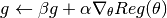Note

When beta is zero, the computed gradient (or its scaled version) will be written to g without using the original data in g (in this case, g need not be initialized).

prox!(reg, r, theta, lambda)

Evaluate the proximal operator, as follows: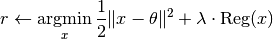This method is needed when proximal methods are used to solve the problem.

In addition, the package also provides a set of generic wrappers to simplify some use cases.

value_and_grad(reg, theta)

Compute and return the regularization value and its gradient w.r.t. theta.

This is a wrapper of value_and_addgrad!.

prox(reg, theta[, lambda])

Evaluate the proximal operator at theta. When lambda is omitted, it is set to 1 by default.

This is a wrapper of prox!.

## Predefined Regularizers¶

The package provides several commonly used regularizers:

### Zero Regularizer¶

The zero regularizer always yields the zero value, which is mainly used to supply an regularizer argument to functions to request it (but you do not intend to impose any regularization).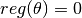immutable ZeroReg <: Regularizer end


### Squared L2 Regularizer¶

This is one of the most widely used regularizer in practice.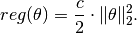immutable SqrL2Reg{T<:FloatingPoint} <: Regularizer
c::T
end

SqrL2Reg{T<:FloatingPoint}(c::T) = SqrL2Reg{T}(c)


### L1 Regularizer¶

This is often used for sparse learning.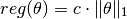immutable L1Reg{T<:FloatingPoint} <: Regularizer
c::T
end

L1Reg{T<:FloatingPoint}(c::T) = L1Reg{T}(c)


### Elastic Regularizer¶

This is also known as L1/L2 regularizer, which is used in the Elastic Net formulation.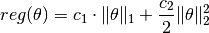immutable ElasticReg{T<:FloatingPoint} <: Regularizer
c1::T
c2::T
end

ElasticReg{T<:FloatingPoint}(c1::T, c2::T) = ElasticReg{T}(c1, c2)